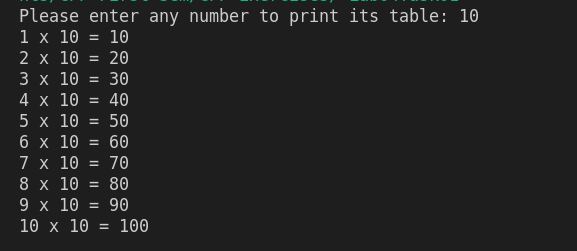Write a C++ program that should ask a user to input an integer number and then displays/prints its table as shown below:cpp programming exercise with solution

#include <iostream>
using namespace std;

int main()
{
int tableNumber;
cout<<"Please enter any number to print its table: ";
cin>>tableNumber;
for (int i = 1; i<= 10; i++)
{
cout<<i<<" x "<<tableNumber<<" = "<<i*tableNumber<<endl;
}

return 0;
}

Using While Loop

#include <iostream>
using namespace std;

int main()
{
int tableNumber;
int i = 1;
cout<<"Please enter any number to print its table: ";
cin>>tableNumber;

while(i<=10)
{
cout<<i<<" x "<<tableNumber<<" = "<<i*tableNumber<<endl;
i++;
}

return 0;
}